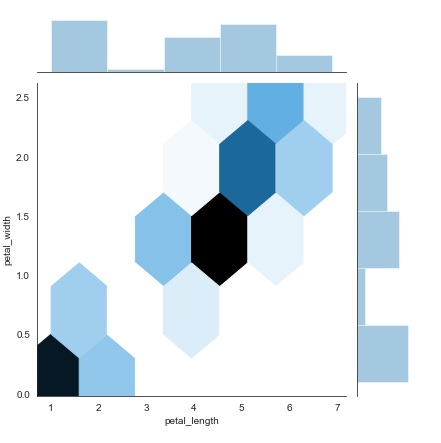# How can Seaborn library be used to display a hexbin plot in Python?

Seaborn is a library that helps in visualizing data. It comes with customized themes and a high level interface. This interface helps in customizing and controlling the kind of data and how it behaves when certain filters are applied to it.

Hexagonal binning can be used in the analysis of bivariate data. This occurs when the data is sparse, i.e. when the data is scattered unevenly. When the data is scattered unevenly, it gets difficult to capture all the data points in a scatterplot.

This is where hexagonal binning comes into play. Let us understand how seaborn library can be used to implement hexagonal binning

## Example

import pandas as pd
import seaborn as sb
from matplotlib import pyplot as plt
sb.jointplot(x = 'petal_length',y = 'petal_width',data = my_df,kind = 'hex')
plt.show()

## Output## Explanation

• The required packages are imported.
• The input data is ‘iris_data’ which is loaded from the scikit learn library.
• This data is stored in a dataframe.